Next: Hamilton's Principle Up: Classical Mechanics Previous: Conditional Variation

# Multi-Function Variation

Suppose that we wish to maximize or minimize the functional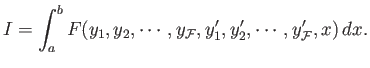(B.61)

Here, the integrand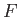is now a functional of the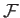independent functions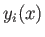, for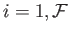. A fairly straightforward extension of the analysis in Section B.5 yieldsseparate Euler-Lagrange equations,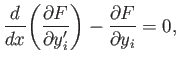(B.62)

for, which determine thefunctions. Ifdoes not explicitly depend on the function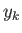then the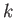th Euler-Lagrange equation simplifies to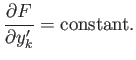(B.63)

Likewise, ifdoes not explicitly depend onthen allEuler-Lagrange equations simplify to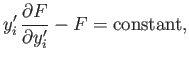(B.64)

for.

Richard Fitzpatrick 2016-01-25